• 原创最近操作系统实习，敲了实现最短寻道优先(SSTF)——磁盘调度管理的代码。题目阐述如下：设计五：磁盘调度管理设计目的：加深对请求磁盘调度管理实现原理的理解，掌握磁盘调度算法。设计内容:通过编程实现不同...

原创
最近操作系统实习，敲了实现最短寻道优先(SSTF)——磁盘调度管理的代码。
题目阐述如下：
设计五：磁盘调度管理
设计目的：
加深对请求磁盘调度管理实现原理的理解，掌握磁盘调度算法。
设计内容:
通过编程实现不同磁盘调度算法。
设定开始磁道号寻道范围，依据起始扫描磁道号和最大磁道号数，随机产生要进行寻道的磁道号序列。
选择磁盘调度算法，显示该算法的磁道访问顺序，计算出移动的磁道总数和平均寻道总数。
常用的磁盘调度算法简介如下，请在以下算法中任意选择两种实现，并对算法性能进行分析对比。
1. 最短寻道优先算法SSTF：该算法选择这样的进程：其要求访问的磁道与当前磁头所在的磁道距离最近，以使每次的寻道时间最短。
2. 扫描算法SCAN：该算法不仅考虑到欲访问的磁道与当前磁道间的距离，更优先考虑的是磁头当前的移动方向。
例如，当磁头正在自里向外移动时，SCAN算法所考虑的下一个访问对象，应是其欲访问的磁道既在当前磁道之外，又是距离最近的。
这样自里向外地访问，直至再无更外的磁道需要访问时，才将磁臂换向为自外向里移动。
3.循环扫描算法CSCAN：CSCAN算法规定磁头单向移动，例如，只是自里向外移动，当磁头移到最外的磁道并访问后，
磁头立即返回到最里的欲访问的磁道，亦即将最小磁道号紧接着最大磁道号构成循环，进行循环扫描。
首先用 rand 函数随机产生磁道号序列，随机选择一磁道号为起点开始寻道。
下一磁道满足在所有磁道中其离当前被访问磁道最近，可用一数组 num_track 存放其他磁道与当前被访问磁道的距离。
在数组 num_track 筛选出数值最小(即离当前被访问磁道最近)的磁道，再以当前磁道为起点，继续计算其他未被访
问磁道与其的距离，再从 num_track 中筛选出数值最小的的磁道来访问......
#include#include#include#include
#define MAX 50 //可访问的最大磁道号
#define N 20 //磁道号数目
int track[N]; //存放随机产生的要进行寻道访问的磁道号序列
int num_track[N]; //记录其他磁道与当前被访问磁道的距离
int total=0; //统计已被访问的磁道号数
int all_track=0; //移动的磁道总数
double aver_track; //平均寻道总数
void SSTF(int order){ //order为track中当前被访问的磁道下标
printf("%d",track[order]);
num_track[order]=-1;
total++; //已被访问磁道号+1
if(total==N){return;
}int i=0;for(i=0;i<=N-1;i++){ //计算其他磁道与当前被访问磁道的距离
if(num_track[i]!=-1){
num_track[i]=abs(track[order]-track[i]);
}
}int min=9999;intx;for(i=0;i<=N-1;i++){ //找出track中与当前被访问磁道距离最短的
if(num_track[i]!=-1){if(num_track[i]
min=num_track[i];
x=i;
}
}
}
all_track+=abs(track[order]-track[x]); //计算当前被访问磁道与下一被访问磁道的距离
SSTF(x);
}intmain(){int i=0;
srand(time(0));
printf("磁道号序列为：");for(i=0;i<=N-1;i++){ //随机产生要进行寻道访问的磁道号序列
track[i]=rand()%(MAX+1);
printf("%d",track[i]);
}
printf("n");
printf("寻道序列为：");
SSTF(rand()%N); //随机选择起点磁道
printf("n移动的磁道总数： %dn",all_track);
printf("平均寻道总数： %0.2lf",(double)all_track/N);return 0;
}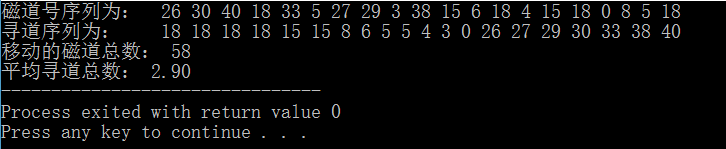(运行结果截图)
17:54:20
2018-05-22
内容来源于网络如有侵权请私信删除

展开全文• 自己写的磁盘调度算法，通俗易懂，其中有先来先服务调度算法最短寻道时间调度算法、电梯调度算法
•  掌握磁盘调度算法，如先来先服务（firstcome first served,FCFS）调度算法最短寻道时间优先（shortest seek timefirst,SSTF）调度算法、扫描（SCAN）调度算法、循环扫描（C-SCAN）调度算法。二、 实验内容设计...
一、  实验目的和要求
1.  了解磁盘调度技术的特点
2.  掌握磁盘调度算法，如先来先服务（firstcome first served,FCFS）调度算法、最短寻道时间优先（shortest seek timefirst,SSTF）调度算法、扫描（SCAN）调度算法、循环扫描（C-SCAN）调度算法。
二、    实验内容
设计模拟实现FCFS、SSTF、SCAN和C-SCAN调度算法的C语言程序。
1.  FCFS算法：根据进程请求访问磁盘的先后次序进行调度。
2.  SSTF算法：要求访问的磁道与当前磁头所在的磁道距离最近，以便每次的寻道时间最短。
3.  SCAN算法：不仅考虑了欲访问的磁道与当前磁道间的距离，更优先考虑的是磁头当前的移动方向。
三、  实验步骤
1.  使用C++语言编译程序。
2.  完成算法代码。
3.  运行程序，算出结果。
四、     实验源程序
代码：
#include <stdio.h>
#include <iostream>
#include <queue>
#include <stack>
#include <set>
#include <string>
#include <cstring>
#include <cmath>
#include <algorithm>
#include <list>
#define MAX 1111
#define INF 0x3f3f3f3f
using namespace std;
typedef struct Disk_Scheduling
{
doubleTrackhao;
doubleTrackshu;

}DKSG;

DKSG ds[MAX];
double track[MAX];
int mark[MAX];

int input(int &n,double &now)
{
int im;
int Min = INF;
printf("请输入所求磁道号个数和开始磁道号(用空格隔开!):\n");
scanf("%d%lf",&n,&now);
printf("请输入%d个磁道号(各磁道号之间用空格隔开!):\n",n);
for(int i=0;i<n; i++){
scanf("%lf",&track[i]);
double temp= fabs(track[i]-now);
if(temp< Min){
Min =temp;
im = i;
}
}
return im;
}

void output(int n)
{
printf("%s  %s\n","被访问的下一个磁道号","横跨磁道数");
for(int i=0;i<n; i++){
printf("%12.2f %16.2f\n",ds[i].Trackhao,ds[i].Trackshu);
}
}

void FCFS()
{
int n;
double now;
input(n,now);
for(int i=0;i<n; i++){
ds[i].Trackhao = track[i];
ds[i].Trackshu = fabs(track[i]-now);
now =track[i];
}
printf("\n先来先服务:\n");
output(n);
}

void SSTF()
{
memset(mark,0,sizeof(mark));
int n;
double now;
int im =input(n,now);
int cnt1,cnt2;
double t1,t2;
for(int i=0;i<n; i++){
int Min =INF;
ds[i].Trackhao = track[im];
ds[i].Trackshu = fabs(track[im]-now);
now =track[im];
mark[im] =1;
for(intj=0; j<n; j++){
if(!mark[j]){
doubletemp = fabs(track[j]-now);
if(temp < Min){
Min = temp;
im = j;
}
}
}
}
printf("\n最短寻道时间优先:\n");
output(n);
}

void SCAN()
{
double retrack[MAX];
int n;
double now;
input(n,now);
sort(track,track+n);
int locate =upper_bound(track,track+n,now) - track;
int t = locate;
int k,r;
for(k=0;k<n-locate; k++){
retrack[k]= track[k+locate];
}
for(r=k; r<n;r++){
retrack[r]= track[--t];
}
for(int i=0;i<n; i++){
ds[i].Trackhao = retrack[i];
ds[i].Trackshu = fabs(retrack[i]-now);
now =retrack[i];
}
printf("\n扫描调度算法:\n");
output(n);
}

void C_SCAN()
{
int n;
double now;
input(n,now);
sort(track,track+n);
int locate =upper_bound(track,track+n,now) - track;
int im;
for(int i=0;i<n; i++){
im =(i+locate)%n;
ds[i].Trackhao = track[im];
ds[i].Trackshu = fabs(track[im]-now);
now =track[im];
}
printf("\n循环扫描调度算法:\n");
output(n);
}

int main()
{
printf("%*s\n",80,"欢迎您！");
int ca = 0;
do{
printf("\n%*s\n",80,"请选择磁盘调度算法或结束程序:");
printf("0、结束程序\n1、先来先服务\n2、最短寻道时间优先\n3、扫描\n4、循环扫描\n");
scanf("%d",&ca);
if(ca == 1)FCFS();
if(ca == 2)SSTF();
if(ca == 3)SCAN();
if(ca == 4)C_SCAN();
printf("\n\n");
}while(ca);
return 0;
}

五、  实验结果

先来先服务调度算法：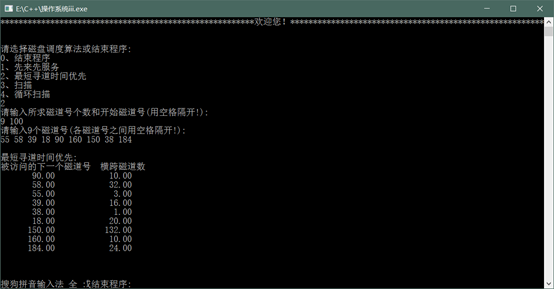最短寻道时间调度算法：扫描调度算法：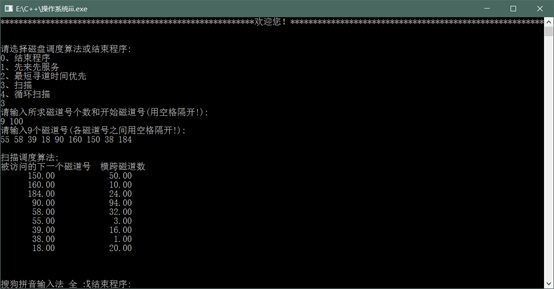循环扫描调度算法：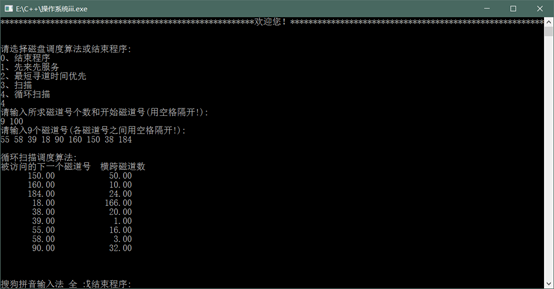展开全文操作系统
• 实现磁盘调度的一些功能，如：先来先服务算法(FCFS)，最短寻道时间优先算法(SSTF)，扫描算法(SCAN)，循环扫描算法(CSCAN)，N步扫描算法(NStepScan)
• 背景：磁盘调度 题目描述 1、对于如下给定的一组磁盘访问进行调度： 请求服务到达 A B C D E F G H I J K L M N 访问的磁道号 30 50 100 180 20 90 150 ...2、要求分别采用先来先服务、最短寻道优先以及电梯调度算法
背景：磁盘调度
题目描述
1、对于如下给定的一组磁盘访问进行调度： 请求服务到达 A B C D E F G H I J K L M N 访问的磁道号 30 50 100 180 20 90 150 70 80 10 160 120 40 110
请求服务到达ABCDEFGHIJKLMN访问的磁道号3050100180209015070801016012040110
2、要求分别采用先来先服务、最短寻道优先以及电梯调度算法进行调度。3、要求给出每种算法中磁盘访问的顺序，计算出平均移动道数。4、假定当前读写头在90号，电梯调度算法向磁道号增加的方向移动。
算法设计
先来先服务算法（FCFS）:
按访问请求到达的先后次序服务。
最短寻道时间优先算法（SSTF）:
优先选择距当前磁头最近的访问请求进行服务
扫描算法（电梯算法）（SCAN）:
当有访问请求时，磁头按一个方向移动，在移动过程中对遇到的访问请求进行服务，然后判断该方向上是否还有访问请求，如果有则继续扫描；否则改变移动方向，并为经过的访问请求服务，如此反复。
代码实现
#include <iostream>
#include <queue>
#include <cmath>
using namespace std;

int request[]{30,50,100,180,20,90,150,70,80,10,160,120,40,110};
const int n = 14;
queue<int> FCFS_order, SSTF_order, SCAN_order;
//queue<int> FCFS_move, SSTF_move, SCAN_move;
int FCFS_num = 0, SSTF_num = 0, SCAN_num = 0;
int beg_loc = 90;//前读写头在90号
char beg_direct = '+';//电梯调度算法向磁道号增加的方向移动。

void C_FCFS() {
int loc = beg_loc;
for (int i = 0; i < n; i++) {
int tem = request[i];
FCFS_order.push(tem);
// FCFS_move.push(abs(loc - tem));
FCFS_num += abs(loc - tem);
loc = tem;
}
}
void C_SSTF() {
int t[n];
for (int i = 0; i < n; i++)
t[i] = request[i];
sort(t, t + n);//排序
int loc = beg_loc;
int pre_loc = beg_loc;
for (int j = 0; j < n; j++){
int min_dis = 9999;
int tem = 0;
for (int i = 0; i < n; i++){
if (abs(t[i] - loc) < min_dis)
min_dis = abs(t[i] - loc), tem = i;
}
loc = t[tem];
SSTF_order.push(loc);
SSTF_num += abs(t[tem] - pre_loc);
pre_loc = loc;
t[tem] = 99999;
}
}
void C_SCAN() {
int t[n];
for (int i = 0; i < n; i++)
t[i]= request[i];
sort(t,t+n);//排序
int loc = 0;
for (int i = 0; i < n; i++)
if (t[i] >= beg_loc) {
loc = i;
break;
}
for (int i = loc; i < n; i++) {
SCAN_order.push(t[i]);
SCAN_num += abs(t[i] - t[i-1]);
}
// SCAN_num-= abs(t[loc] - t[loc - 1]);//初始位置没有移动
SCAN_num += abs(t[n-1] - t[loc - 1]);//转头回来
for (int i = loc-1; i >= 0; i--) {
SCAN_order.push(t[i]);
SCAN_num += abs(t[i] - t[i + 1]);
}

}
int main()
{
C_FCFS();
C_SSTF();
C_SCAN();

cout << "FCFS平均" << (double)FCFS_num / n << "\t顺序:";
while (FCFS_order.size()) {
cout << FCFS_order.front() << " ";
FCFS_order.pop();
}
cout << "\nSSTF平均" << (double)SSTF_num / n << "\t顺序:";
while (SSTF_order.size()) {
cout << SSTF_order.front() << " ";
SSTF_order.pop();
}
cout << "\nSCAN平均" << (double)SCAN_num / n << "\t顺序:";
while (SCAN_order.size()) {
cout << SCAN_order.front() << " ";
SCAN_order.pop();
}

return 0;
}

/*
先来先服务算法（FCFS）:按访问请求到达的先后次序服务。
最短寻道时间优先算法（SSTF）:优先选择距当前磁头最近的访问请求进行服务
扫描算法（电梯算法）（SCAN）:
当有访问请求时，磁头按一个方向移动，在移动过程中对遇到的访问请求进行服务，
然后判断该方向上是否还有访问请求，如果有则继续扫描；
否则改变移动方向，并为经过的访问请求服务，如此反复。

*/

运行结果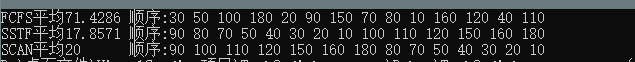展开全文• 磁盘移臂调度过程模拟设计--电梯算法最短寻道时间优先算法
• 最短寻道时间优先算法（SSTF） SSTF问题描述：SSTF算法选择调度处理的磁道是与当前磁头所在磁道距离最近的磁道，以使每次的寻找时间最短。当然，总是选择最小寻找时间并不能保证平均寻找时间最小，但是能提供比FCFS...
目录
1，最短寻道时间优先算法（SSTF）
2，扫描算法（又称电梯算法）

1，最短寻道时间优先算法（SSTF）
SSTF问题描述：SSTF算法选择调度处理的磁道是与当前磁头所在磁道距离最近的磁道，以使每次的寻找时间最短。当然，总是选择最小寻找时间并不能保证平均寻找时间最小，但是能提供比FCFS算法更好的性能。这种算法会产生“饥饿”现象。 1、算法思想：优先选择距当前磁头最近的访问请求进行服务，主要考虑寻道优先。 2、优点：改善了磁盘平均服务时间。 3、缺点：造成某些访问请求长期等待得不到服务。  本算法是对输入的磁道首先进行非递减排序，然后判断当前磁头所在的磁道是否在将要寻找的磁道中，分别进行最短寻道时间计算。（如下图示，表示SSTF示意图）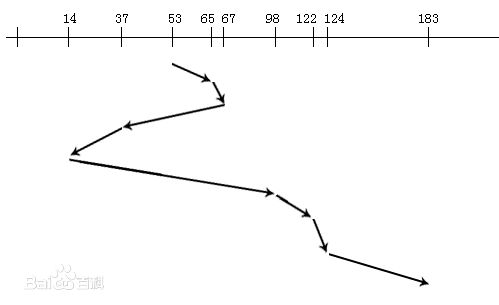代码实现：
//最短寻道时间优先SSTF
#include "pch.h"
#include <iostream>
using namespace std;
//快速排序
int Partition(int *p, int low, int high)
{
int i = low, j = high, pivot = p[low];
while (i < j)
{
while (i < j&&p[j] >= pivot)
{
j--;
}
if (i < j)
{
p[i] = p[j];
i++;
}
while (i < j&&p[i] <= pivot)
{
i++;
}
if (i < j)
{
p[j] = p[i];
j--;
}
}
p[i] = pivot;
return i;
}
void QuickSort(int *q, int left, int right)
{
if (left < right)
{
int pivotpos = Partition(q, left, right);
QuickSort(q, left, pivotpos - 1);
QuickSort(q, pivotpos + 1, right);
}

}
int main()
{
int count = 0;//输入磁盘请求的个数
int currentStair;//当前所在的磁道
int n;
int temp_1, temp_2;
int size = 0;//步数计数变量
int temp_3, temp_4;
cout << "请输入要寻到的数量:" << endl;
cin >> count;
n = count;
int *arr = new int[count];
cout << "请输入要寻得磁道:" << endl;
for (int i = 0; i < count; i++)
{
cin >> arr[i];//输入楼梯数
}
cout << "please input currentstars:" << endl;
cin >> currentStair;
QuickSort(arr, 0, count - 1);
for (int i = 0; i < count; i++)//当前磁道在要寻磁道中
{
if (currentStair == arr[i]) {
currentStair = arr[i];
temp_1 = i - 1;
temp_2 = temp_1 + 1;
while (temp_1 >= 0 && temp_2 < count)
{
if (abs(currentStair - arr[temp_1]) < abs(arr[temp_2] - currentStair))
{
size += abs(currentStair - arr[temp_1]);
currentStair = arr[temp_1];
if (temp_1 > 0)
temp_1 -= 1;
if (currentStair == arr[temp_1])
{
while (temp_2 < count)
{
size += abs(arr[temp_2] - currentStair);
currentStair = arr[temp_2];
temp_2++;
}
}
}
else {
size += abs(arr[temp_2] - currentStair);
if (temp_2 < count)
temp_2 += 1;
if (currentStair == arr[temp_2])
{
while (temp_1 > 0)
{
size += abs(arr[temp_1] - currentStair);
currentStair = arr[temp_1];
temp_1--;
}
}

}
}
}
}
for (int h = 0; h < count; h++)//当前所在的位置不在要寻的磁道中
{
if (currentStair > arr[h - 1] && currentStair < arr[h])//定位当前的位置
{
temp_3 = h - 1;
temp_4 = h;
while (temp_3 >= 0 && temp_4 < count)
{
if (abs(currentStair - arr[temp_3]) < abs(arr[temp_4] - currentStair))
{
size += abs(currentStair - arr[temp_3]);
currentStair = arr[temp_3];
if (temp_3 > 0)
temp_3 -= 1;
if (currentStair == arr[temp_3])
{
while (temp_4 < count)
{
size += arr[temp_4] - currentStair;
currentStair = arr[temp_4];
temp_4++;
}
}
}
else {
size += abs(arr[temp_4] - currentStair);
currentStair = arr[temp_4];
if (temp_4 < count)
temp_4 += 1;
if (currentStair == arr[temp_4])
{
while (temp_3 > 0)
{
size += arr[temp_3] - currentStair;
currentStair = arr[temp_3];
temp_3--;
}
}

}
}
}
else
if (currentStair < arr)
{
int i = 0;
while (i < count)
{
size += abs(arr[i] - currentStair);
currentStair = arr[i];
i++;
}
}
else
if (currentStair > arr[count - 1])
{
int j = count - 1;
while (j > 0)
{
size += abs(arr[j] - currentStair);
currentStair = arr[j];
j--;
}
}

}
int average = size / count;
cout << "最少寻磁道数是:"<<size<<"平均寻磁道数是："<<average<< endl;
}

2，扫描算法（又称电梯算法）
扫描算法问题描述：SCAN算法在磁头当前移动方向上选择与当前磁头所在磁道距离最近的请求作为下一次服务的对象。由于磁头移动规律与电梯运行相似，故又称为电梯调度算法。SCAN算法对最近扫描过的区域不公平，因此，它在访问局部性方面不如FCFS算法和SSTF算法好。算法思想：当设备无访问请求时，磁头不动；当有访问请求时，磁头按一个方向移动，在移   动过程中对遇到的访问  请  求   进行服务，然后判断该方向上是否还有访问请求，如果有则继续扫描；否则改变移动方向，并为经过的访问请求服务，如此反复 。优点：克服了最短寻道优先的缺点，既考虑了距离，同时又考虑了方向。（如下图表示SCAN图示）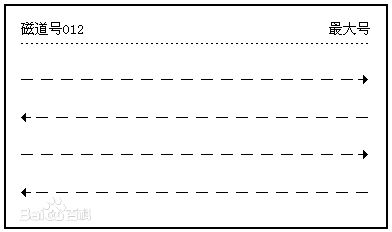代码实现：
import java.util.Scanner;
public class Test_louti {
//本算法我假设楼梯最大到200层，
public static void main(String[] args) {
Scanner input =new Scanner(System.in);
int []arr=new int;//200楼电梯
System.out.println("请输入你要输入的楼层数：");
int number=input.nextInt();//number记录当前所在楼梯
int b=0;
System.out.println("请输入楼梯:");
for(int i=0;i<=200;i++)
{
if(b<number){
int num=input.nextInt();
arr[num]=num;//记住楼梯,从小到大
b++;
i++;
}
if(b==number)
break;
}
System.out.println("请输入当前楼梯号码：");
int currentScan=input.nextInt();//当前楼梯
int i=currentScan,team=0,j=0,a=currentScan;
arr[currentScan]=currentScan;
while(i<200)//i记住当前判断的楼梯号码
{
if(arr[i]!=0)
{
team+=(arr[i]-arr[currentScan]);
currentScan=i;
j=i;//记住最后的楼梯号码
}
i+=1;
}
//下半层
while(a>0)
{
if(arr[a]!=0)
{
team+=Math.abs((arr[a]-arr[j]));
j=a;
}
a-=1;
}
System.out.println("总的移动量是："+team);
}
}


展开全文• 一、设计目的： 加深对请求磁盘调度管理实现原理的理解，掌握磁盘调度算法中的最短寻道优先算法。二、设计内容通过编程实现磁盘调度中最短寻道优先算法。设定开始磁道号寻道范围，依据起始扫描磁道号和最大磁道号数...
• 最近在腾讯的笔试题中看到最短寻道时间的题目，然后就去看了下相关资料，了解了下SSTF算法的实现（原理就是优先访问离当前读写头最近的位置） 例如：磁盘访问序列为：35，12，73，230，80，20，310，120 读写头...
• 磁盘移臂调度过程模拟设计--先来先服务法、最短寻道时间优先算法
• 最短寻找时间优先调度算法总是从等待访问者中挑选寻找时间最短的那个请求先执行的，而不管访问者到来的先后次序。比如现有需要读取的数据的柱面次序为：35 12 73 230 80 20 310 120，初始柱面为65号，当65操作结束后...操作系统 c++
• 最近操作系统实习，敲了实现最短寻道优先（SSTF）——磁盘调度管理的代码。 题目阐述如下： 　设计五：磁盘调度管理 设计目的： 加深对请求磁盘调度管理实现原理的理解，掌握磁盘调度算法。 设计内容: 通过...
• 最短寻道时间优先算法(SSTF) 扫描算法(SCAN) 先来先服务算法(FCFS) 循环扫描算法(CSCAN)....
• 基于java图形界面开发出“Stephen的磁盘调度mini模拟器”，实现出先来先服务&最短寻道&扫描算法&循环扫描算法的执行效果。
• 若干个等待访问磁盘者依次要访问的柱面编号为：80,40,74,14,60,31,61，假设每移动一个...（2）若采用最短寻道时间优先算法调度，求移动臂的移动次序和所花费的寻找时间。 解： （1）SCAN算法调度 下一...
• SSTF算法选择调度处理的磁道是与当前磁头所在磁道距离最近的磁道，以使每次的寻找时间最短。当然，总是选择最小寻找时间并不能保证平均寻找时间最小，但是能提供比FCFS算法更好的性能。这种算法会产生“饥饿”现象。...
• 学号 P71514032 专业 计算机科学与技术 姓名 实验日期 2017/12/7 教师签字 成绩 实验报告 实验名称 磁盘调度先来先服务策略 最短寻道策略 实验目的 磁盘... 最短寻道时间优先算法 最短寻道时间优先算法要求访问的磁道
• * 代码功能：使用先来先服务、最短寻道优先、电梯调度方法进行调度。 * 求出平均移动道数。当前读写头在90号，向磁道号增加的方向移动。 * */ public class DiskScheduling { public static void main(String ...java
• 请珍惜小编劳动成果，该文章为小编原创，转载请注明出处。 该算法选择这样的进程，其要求访问的磁道与当前磁头所在的磁道距离最近，以使每次的...//最短寻道时间优先 public class SSTF { private int sh...
• 先来先服务(FCFS)、最短寻道时间优先(SSTf)、扫描算法(SCAN)、循环扫描算法(CSCAN)最后有运行截图。#include#include#include#includeint a; //当前磁道号int b; //磁头运动方向int c; //要访问的柱面号int num;...
• 1、对于如下给定的一组磁盘...2、要求分别采用先来先服务、最短寻道优先以及电梯调度方法进行调度。 3、要求给出每种算法中磁盘访问的顺序，计算出平均移动道数。 4、假定当前读写头在90号，向磁道号增加的方向移动。
• 1、对于如下给定的一组磁盘...2、要求分别采用先来先服务、最短寻道优先以及电梯调度方法进行调度。 3、要求给出每种算法中磁盘访问的顺序，计算出平均移动道数。 4、假定当前读写头在90号，向磁道号增加的方向移动。
• 操作系统实验之磁盘调度算法模拟（最短寻道时间优先SSTF 和 扫描算法SCAN） 最短寻道时间优先SSTF 要求每次访问的磁道与当前磁头所在的磁道距离最近、 也就是每次访问距离磁头最近的磁道 扫描算法SCAN 由里向外地...操作系统试验 SCAN
• 最短寻道时间优先（SSTF）和扫描（SCAN）算法。理解各调度算法的工作原理 对给出的任意的磁盘请求序列、计算平均寻道长度；要求可定制磁盘请求序列长度、磁头起始位置、磁头移动方向。 测试：假设磁盘访问序列：98，...操作系统实验
• 选择这样的进程，其要求访问的磁道与当前磁头所在的磁道距离最近，以使每次的寻道时间最短#include <malloc.h> #include<stdio.h> #include<math.h> #include <limits.h> typedef struct track{ int column; ...
• 磁盘调度算法（先来先服务。最短寻道时间算法） 操作系统课程设计操作系统课程设计
• 先简单介绍一下这几种算法： 1、先来先服务：最早提交最早访问。 例如：磁盘访问序列：98...2、最短寻道时间优先：离读写头最近的最早访问。 例如：磁盘访问序列：98，183，37，122，14，124，65，67。 读写头起...先来先服务 操作系统
• 由于有时候这些进程的发送请求的速度比磁盘响应的还要快，因此我们有必要为每个磁盘设备建立一个等待队列，常用的磁盘调度算法有以下四种：先来先服务算法(FCFS)，最短寻道时间优先算法(SSTF)，扫描算法(SCAN)，循环......